Latest Banking jobs   »

# Quantitative Aptitude Quiz For Bank of Baroda AO 2023 -4th March

Direction (1-5): The line graph given below shows the production and consumption (in quintal) of Rice in 5 different years of a village. Study the graph carefully and answer the following questions.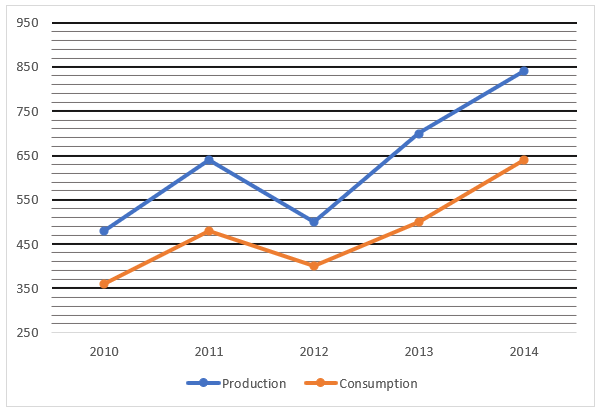Q1. What is the average consumption of Rice all over the years?
(a) 445 Quintals
(b) 254 Quintals
(c) 316 Quintals
(d) 476 Quintals
(e) 378 Quintals

Q2. Find the production of rice in 2011 is what percentage more/less than that in 2012?
(a) 28%
(b) 33%
(c) 30%
(d) 25%
(e) 23%

Q3. What is the respective ratio between the consumption of rice in 2012 and 2013 together to the production of rice in 2010 and 2011 together?
(a) 45 : 53
(b) 45 : 56
(c) 12 : 17
(d) 15 : 17
(e) 30 : 53

Q4. If in 2015 the ratio between the production and consumption of rice is 7 : 5 and total production of rice is 280 Quintal, then consumption of rice in 2015 is how much more/less than previous year?
(a) 150 quintals
(b) 180 quintals
(c) 140 quintals
(d) 120 quintals
(e) 220 quintals

Q5. In which year percentage of consumption of rice is maximum with respect to production?
(a) 2012
(b) 2014
(c) 2011
(d) 2013
(e) 2010

Directions (6-10): What value should come in place of (?) in the following questions?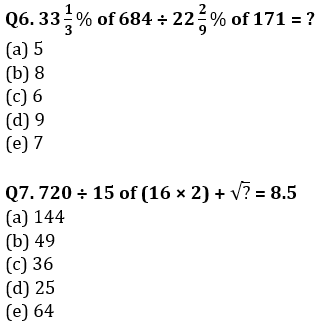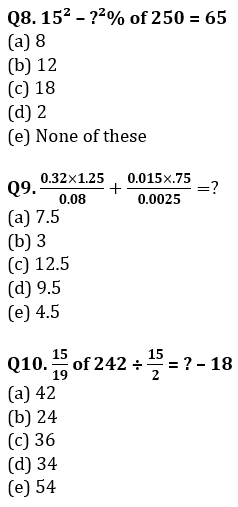Directions (11-15): There are two equations in x and y are given in each question. You have to solve both the equations and give answer accordingly:
(a) if x > y
(b) if x < y
(c) if x = y or relation between x & y cannot be established
(d) x ≥ y
(e) x ≤ y
Q11. I. x² + 21x – 100 = 0
II. y² – 13y + 36 = 0
Q12. I. 2x² – 8x – 24 = 0
II. 3y² – 9y – 12 = 0
Q13. I. 3y² – 42y + 72 = 0
II. 4x² – 44x + 40 = 0
Q14. I. 9x² – 72x + 144 = 0
II. 25y² – 240y + 576 = 0
Q15. I. x² – 96x + 2268 = 0
II. y² = 1764

Solutions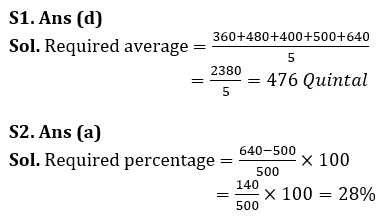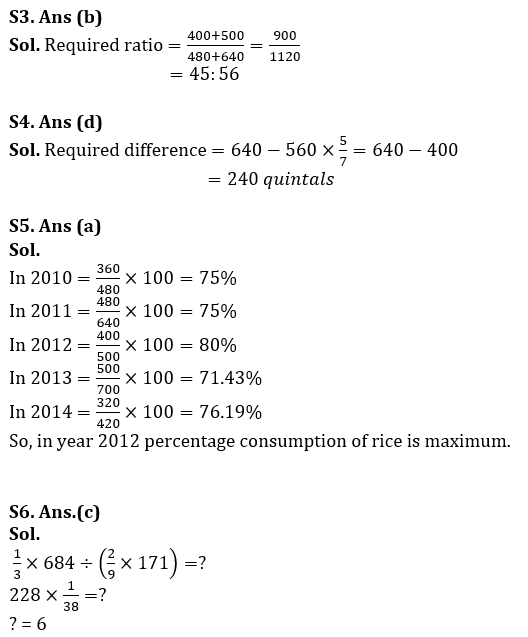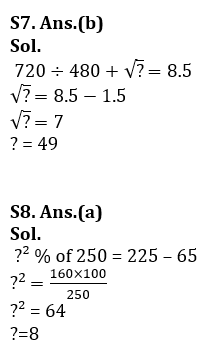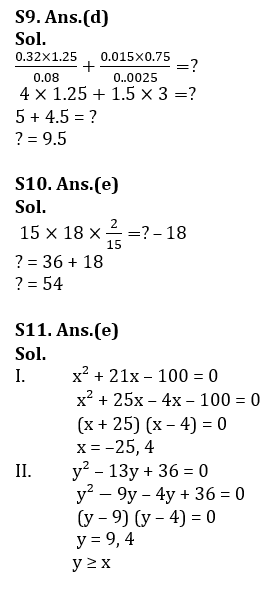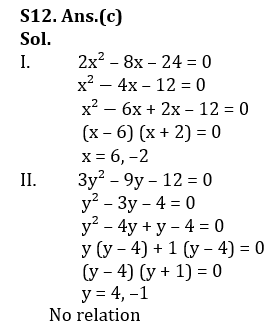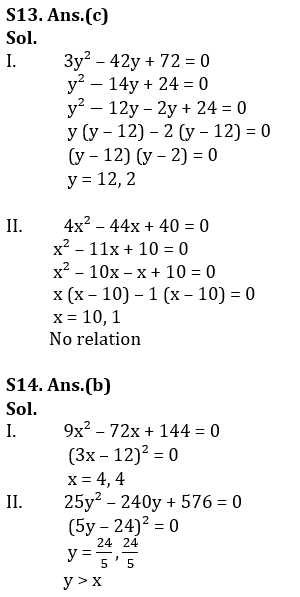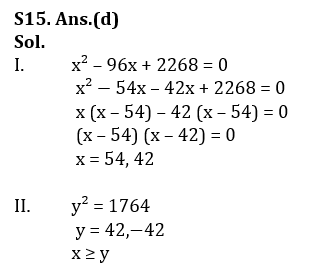## FAQs

### What is the selection process of Bank of Baroda AO 2023?

The selection process of Bank of Baroda AO consists of Online exam and Interview

#### Congratulations!Union Budget 2023-24: Free PDF